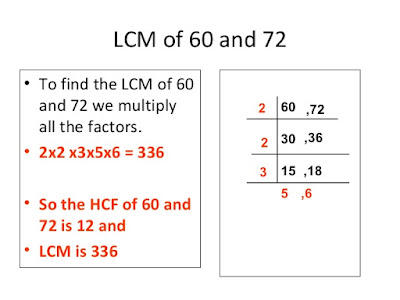## Search Here

### PRIME FACTORIZATION : Finding HCF & LCM with Prime Factorization

We want to find the HCF and LCM of the numbers 60 and 72.
Start by writing each number as a product of its prime factors.60 = 2 × 2 × 3 × 5
72 = 2 × 2 × 2 × 3 × 3
To make the next stage easier, we need to write these so that each new prime factor begins in the same place:

60 = 2 × 2 × 3 × 5
72 = 2 × 2 × 2 × 3 × 3

All the "2"s are now above each other, as are the "3"s etc. This allows us to match up the prime factors. The highest common factor is found by multiplying all the factors which
appear in both lists:

So the HCF of 60 and 72 is 2 × 2 × 3 which is 12.

The lowest common multiple is found by multiplying all the factors which appear in either  list:
So the LCM of 60 and 72 is 2 × 2 × 2 × 3 × 3 × 5 which is 360.